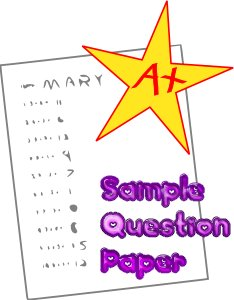# IPU BCA Semester 3: Computer Architecture - End Term Question Paper (2014)

END TERM EXAMINATION
(2014)
THIRD SEMESTER [BCA]

## COMPUTER ARCHITECTURE

[BCA-205]Time: 3 hrs.                           M.M.: 75

Note: Attempt any five questions including Q. No.1 which is compulsory. Select one question from each unit.

Question 1:
(a) What is a three-state buffer? Explain its working. (2)

(b) What is insert operation? Give one example.

(c) A digital computer has a common bus system for 16 registers of 32 bits each. The bus is constructed with multiplexes. (3)
(i) How many selection inputs are there in each multiplexer?
(ii) What size of multiplexers are needed?
(iii) How many multiplexers are there in the bus?

(d) Explain direct and indirect addressing modes using examples.

(f) What are 3 types of pipeline conflicts? Discuss them in brief. (3)

(g) Design 2-bit by 2-bit array multiplier.

(h) What is the need of input-output interface? (2)

(i) Write a short note on memory hierarchy. (2)

(j) An instruction is stored at location 300 with its address field at location 301. The address field has the value 400. A processor register RI contains the number 200. Evaluate the effective address if the addressing mode of the instruction is
(i) direct
(ii) immediate
(iii) relative and
(iv) indirect.

UNIT I

Question 2:
(a) What is a bus ? Design and explain a bus system using multiplexers regarding 4 registers of 4 bit each.                                                                                                               (7.5)
(b) Draw and explain the flowchart for interrupt cycle. (5)

Question 3:
(a) Starting from an initial value of R =  1 1 1 1 0 1 1 0, determine the sequence of binary values of R after a logical shift-left, followed by a circular shift-right, followed by a logical shift-right and a circular shift-left.

(b) What are input-output instructions? Explain all input-output instructions.        (6.5)

UNIT II

Question 4:

(a) Write a program to evaluate the arithmetic X= (A-B+C*D*E-F)/(G+H*K). (8)
(i) Using a general register computer with 3-address instruction.
(ii) Using a general register computer with 2-address instructions.
(iii) Using an accumulator type computer with 1-address instructions.
(iv) Using a stack organized computer with zero address operation instructions.

(b) What is pipeline/pipeline processing? Discuss with the help of suitable example.           (4.5)

Question 5:

(b) Write a short note on memory interleaving. (4)

UNIT III

Question 6:

(a) Show the step-by-step multiplication process using Booth algorithm when the following binary numbers are multiplied. Assume 4-bit registers that hold signed numbers:
(i) (+5) × (-5) ×(-3) (5)
(ii) Use 4 bits for representation.

(b) What is asynchronous data transfer? Discuss asynchronous data transfer using-
(i) strobe control and
(ii) handshaking with timing and block diagrams.      (7.5)

Question 7:
(a) Show the contents of registers E,A,Q and SC during the process of division of
(i) 10110011 by 1001
(ii) 11110000 by 0011. (use a divided of 8 bits).       (5)

(b) Explain DMA. Discuss DMA controller using suitable block diagrams.     (7.5)

UNIT IV

Question 8:
(a) Explain associative memory with suitable block diagram and suitable example.     (6.5)
(b) Giving suitable block diagram differentiate between RAM and ROM.      (6)

Question 9: What is memory mapping? Explain various types of memory mapping using suitable block diagrams.                                                                                     (12.5)

This post first appeared on IPU BCA, BBA, MCA, MBA Examination Papers And Notes, please read the originial post: here

# Share the post

IPU BCA Semester 3: Computer Architecture - End Term Question Paper (2014)

×